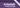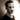TypeclassesBack in ... purple?

It's been a while, but welcome back to yet another installment in our read-through of Haskell Programming from First Principles. This time we're looking at typeclasses.

## What are typeclasses?

Typeclasses are like interfaces to your data. The description that I find the most intuitive is that a typeclass is a set of functions that must be implemented for your type to be an 'instance' of a typeclass. In classic OO-terms, this is very much like an interface; a contract that you must fulfill to have your type be able to stand in for this interface. In this way, typeclasses are a vehicle for ad-hoc or constrained polymorphism, and let you write code that operates on a more generic set of types than concrete implementations.

Typeclasses allow us to generalize over a set of types and to write functions that can abstract over concrete implementations. For instance: if all a function does with one of its input parameters is test it for equality, then the `Eq` typeclass contains all the functionality we need, and we can make that function take any type that implements the `Eq` typeclass. Similarly, all numeric literals and their various types implement the typeclass `Num`, which defines a set of standard numeric operations, allowing functions to work on any numeric value.

To get a better feel for how this works, let's look at some of the basic typeclasses and some implementations!

## `Eq`

`Eq`, the typeclass for equality. A very simple typeclass that will let you decide whether two items are the same or not.

The definition is as follows:

``````class Eq a where
(==) :: a -> a -> Bool
(/=) :: a -> a -> Bool``````

Pretty simple, right? All it needs is a definition for `(==)` and one for `(/=)`, and even better, the minimal complete definition --- that is, the least we need to implement to have a complete definition --- is just one of the two functions. The one we don't define will default to being the negation of the one we do define.

Most data types you'll work with implement this one already, so you don't need to worry much about it. Interestingly, because Haskell deals with structural equality, whether a tuple is an instance of `Eq` or not, depends on its components. If all the components can be compared for equality, then so can the tuple. This also applies to lists and a range of other data structures.

### Writing an instance of the `Eq` typeclass

As an aside, here's how you'd write your own instance of the `Eq` typeclass. It's a simple instance to write, but that makes it easier to learn from. Let's look at a basic example using a simple type we define, `Bool2`:

``````data Bool2 = No | Yes

instance Eq Bool2 where
Yes == Yes = True
No  == No  = True
_   == _   = False``````

So to create an instance, we put `instance`, the name of the typeclass, the name of the type to implement it for, and the `where` keyword. What follows is an implementation of the functions required for the typeclass. As mentioned above, the minimal complete definition of the `Eq` typeclass only requires one of the functions to be defined, so this is a valid implementation.

## `Num`

The `Num` typeclass is one we've already looked at quite a bit previously, so we're not going to dwell on it for too long. It's a typeclass implemented by most of the numeric types, and the definition is as follows:

``````class Num a where
(+) :: a -> a -> a
(*) :: a -> a -> a
(-) :: a -> a -> a
negate :: a -> a
abs :: a -> a
signum :: a -> a
fromInteger :: Integer -> a``````

### `Fractional`

There are various other typeclasses related to `Num`, but let's look at `Fractional`, a subtype of `Num`:

``````class (Num a) => Fractional a where
(/) :: a -> a -> a
recip :: a -> a
fromRational :: Rational -> a``````

This typeclass requires its type argument, `a`, to have an instance of `Num`. This means that a `Fractional` is a specialized `Num` and can be used anywhere a `Num` can, but also specifies an extra set of functionality that is not required of the regular `Num` typeclass.

## `Ord`

This typeclass takes care of ordering things, and as such, has a definition concerned with orders and comparisons:

``````class Eq a => Ord a where
compare :: a -> a -> Ordering
(<) :: a -> a -> Bool
(>=) :: a -> a -> Bool
(>) :: a -> a -> Bool
(<=) :: a -> a -> Bool
max :: a -> a -> a
min :: a -> a -> a``````

Note that it has a typeclass constraint of `Eq`. If you're going to be comparing things, you're going to have to be able to tell whether they are equal or not. These functions all work the way you'd expect them to.

Ord instances (when derived automatically) rely on the way the data type is defined. Looking back on our `Bool2` data type from earlier (`data Bool2 = No | Yes`), `Yes` will be greater than `No` because it is defined later. This is similar to how enums work in the C-familiy of languages where they're usually just fancy names for successive integers starting at an arbitrary point and incrementing by one for each entry. Naturally, if you want to change the ordering, you can, but you're going to have to implement the typeclass yourself.

## `Enum`

The `Enum` typeclass is quite different (yet weirdly similar) to most other things known as enums in other programming languages (at least in my experience). In Haskell, the `Enum` typeclass is for types that are enumerable, that have known predecessors and successors. What does this mean? It means you can use instances of this typeclass to generate ranges and lists.

Definition:

``````class Enum a where
succ :: a -> a
pred :: a -> a
toEnum :: Int -> a
enumFrom :: a -> [a]
enumFromThen :: a -> a -> [a]
enumFromTo :: a -> a -> [a]
enumFromThenTo :: a -> a -> a -> [a]``````

The most obvious instance of this typeclass would be something like integers, where `succ 4` is `5` and `pred 3` is `2`. Similarly, characters can be enumerated just as well, as can ordered data types like `Bool`.

Some of the function names listed above are a bit confusing (`enumFromThenTo` anyone?), but when you look at it in context it makes more sense. For instance,

``````enumFromThenTo 1 10 100
-- yields [1,10,19,28,37,46,55,64,73,82,91,100]``````

Where this becomes more interesting is when you see that you can use them in list comprehensions and figure out that there's alternative ways of using these methods. Here's the equivalent written as a list comprehension instead:

``````[1, 10 .. 100]
-- yields [1,10,19,28,37,46,55,64,73,82,91,100]``````

## `Show`

`Show` is a typeclass that provides human-readable string-representations of structured data. GHCi uses this to create `String` values that it can print in the terminal. In fact, to have a type be printed in the terminal, it must implement `Show`.

``````class Show a where
showsPrec :: Int -> a -> ShowS
show :: a -> String
showList :: [a] -> ShowS``````

Unless you're writing this for your own data structures, you don't need to worry much about this one. It's already implemented for all basic types. The minimal complete definition requires either `show` or `showsPrec`.

## Definitions

We've covered a lot of ground here, so let's take a step back and look at some definitions for this chapter.

Typeclass inheritance
When a typeclass has a superclass. This means that only data types that implement the super class can have an instance of the subclass. Going back to the `Num` and `Fractional` typeclasses: Every `Fractional` is also a `Num`, but not every `Num` is a `Fractional`. This makes `Num` a superclass of `Fractional`.
Instance
An instance is the definition of how a typeclass should work for a given type. Instances must be unique for a given combination of typeclass and type, i.e. you cannot have two different instances of a typeclass `T` for a single type `a`.
Derived instances
Some typeclasses are so obvious or common that they can be automatically implemented for a data type. Automatically deriving typeclasses like this is done by specifying it after the data declaration: `data MyType = A | B deriving (Eq, Show)`Thomas Heartman is a developer, writer, speaker, and one of those odd people who enjoy lifting heavy things and putting them back down again. Preferably with others. Doing his best to gain and share as much knowledge as possible.